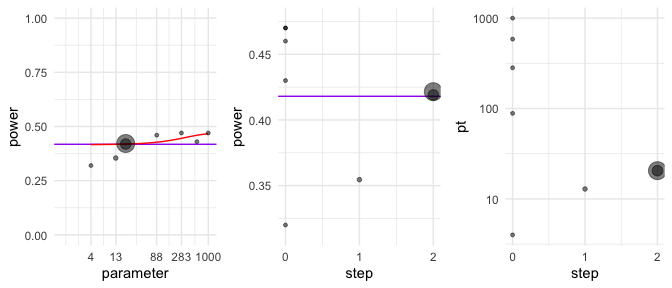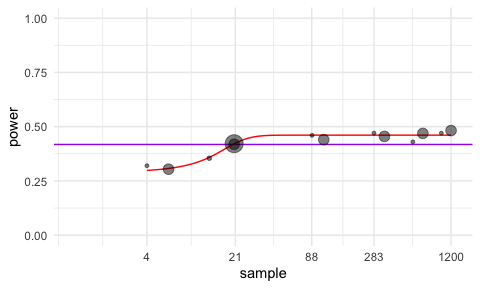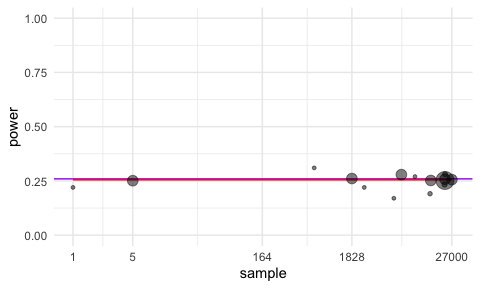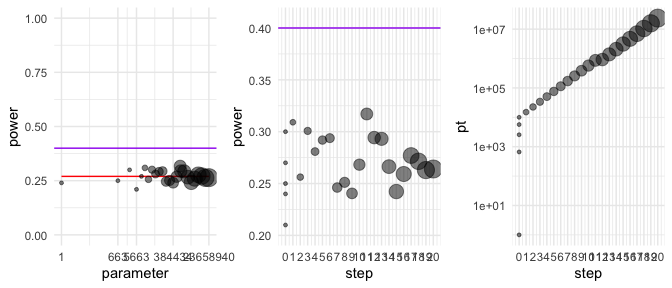# Introduction

The main PUMP vignette covers all of the main functions of the package. This vignette focuses on one specific function: pump_sample(), which calculates required sample sizes at various levels of an RCT design. This function requires extra explanation because of both its unique interpretation and behavior. First, this vignette discusses how the user should interpret the output of a call to pump_sample(). Second, this vignette discusses some challenges the user might face when using the function.

# Intepreting sample size calculations

Let's start with a case of calculating the sample size for a 3-level model. To demonstrate some of the challenges of calculating sample size, we start with calculating power for a given set of parameters, and then try to recover those parameters.

p <- pump_power(
d_m = "d3.1_m3rr2rr",
MTP = "HO",
nbar = 50,
K = 15,
J = 20,
M = 3,
MDES = rep(0.125, 3),
Tbar = 0.5, alpha = 0.05,
numCovar.1 = 1, numCovar.2 = 1,
R2.1 = 0.1, R2.2 = 0.1,
ICC.2 = 0.2, ICC.3 = 0.2,
omega.2 = 0.2, omega.3 = 0.2,
rho = 0.5, tnum = 100000
)
MTP D1indiv D2indiv D3indiv indiv.mean min1 min2 complete
None 0.56213 0.56524 0.56428 0.5638833
HO 0.41618 0.41911 0.41886 0.4180500 0.58365 0.39567 0.31228

Our individual power using the Holm procedure is estimated as 0.418. First, let's try to recover the original parameter $$K$$, the number of districts, given the power we just found.

K <- pump_sample(
d_m = "d3.1_m3rr2rr",
typesample = "K",
MTP = "HO",
target.power = target_power,
power.definition = "D1indiv",
J = 20,
nbar = 50,
M = 3,
MDES = 0.125,
Tbar = 0.5, alpha = 0.05,
numCovar.1 = 1, numCovar.2 = 1,
R2.1 = 0.1, R2.2 = 0.1,
ICC.2 = 0.2, ICC.3 = 0.2,
omega.2 = 0.2, omega.3 = 0.2, rho = 0.5
)
MTP Sample.type Sample.size D1indiv.power
HO K 16 0.4165

We recover a similar value K = , but not the exact value of the original parameter. A small discrepancy from the original parameter is unsurprising given two features of the package. First, PUMP power calculations rely on simulations, so the returned values are estimates with some uncertainty. The randomness inherent to simulations means that repeating the same power calculation with the same set of parameters might result in slightly different power estimates. In this example, we have two sources of uncertainty: uncertainty in what the actual power is (from our first call), and uncertainty in the power calculations of pump_sample().

Second, the search algorithm finds a sample size which is within a certain tolerance of the target power, give or take this estimation uncertainty. The default tolerance is $$0.01$$, meaning the algorithm will return a value of $$K$$ that has an estimated power within 1% of our target power.

These two facts highlight how the output should be interpreted: pump_sample finds one possible sample size that would result in a power within the given tolerance of our target power, give or take the estimation error. For computational efficiency, pump_sample also usually is run with fewer simulation iterations, meaning its estimates are bit more noisy. We therefore advise checking pump_sample's work: given an initial sample size value, it is worth plugging it back into pump_power with a high degree of precision (by increasing tnum, the number of test statistics draws) to verify the intended power. One easy way to verify the power is to use the update() function. We feed in the sample size object and specify that we now want to run a power calculation, and can also provide any other parameters that we would like to update. Here, we override the default $$tnum$$ for pump_power() to increase precision.

p <- update(K, type = "power", tnum = 100000)
MTP D1indiv D2indiv D3indiv indiv.mean min1 min2 complete
None 0.59761 0.59543 0.59621 0.5964167
HO 0.45930 0.45677 0.45735 0.4578067 0.62488 0.43872 0.34743

We also could find that other values of $$K$$, including smaller values, would result in a very similar power, so the user should explore different possible values near the output to get a better sense of the variation in power. This idea will be explored in the next section.

# Exploring a range of sample size values

Now let's turn to determining the necessary number of schools $$J$$, given a fixed $$K$$.

J1 <- pump_sample(
d_m = "d3.1_m3rr2rr",
typesample = "J",
MTP = "HO",
target.power = target_power,
power.definition = "D1indiv",
K = 15,
nbar = 50,
M = 3,
MDES = 0.125,
Tbar = 0.5, alpha = 0.05,
numCovar.1 = 1, numCovar.2 = 1,
R2.1 = 0.1, R2.2 = 0.1,
ICC.2 = 0.2, ICC.3 = 0.2,
omega.2 = 0.2, omega.3 = 0.2,
rho = 0.5
)
MTP Sample.type Sample.size D1indiv.power
HO J 21 0.4215

Our calculated $$J$$ is only slightly off from our original input 20. Let's explore more information about this sample size calculation. First, we can print out information about the search path the algorithm took to arrive at the final answer.

search_path(J1)
#>   step MTP target.power         pt          dx    w     power       delta
#> 1    0  HO        0.418    4.00000          NA  100 0.3200000 -0.09800000
#> 2    0  HO        0.418   88.46708          NA  100 0.4600000  0.04200000
#> 3    0  HO        0.418  282.62278          NA  100 0.4700000  0.05200000
#> 4    0  HO        0.418  586.46708          NA  100 0.4300000  0.01200000
#> 5    0  HO        0.418 1000.00000          NA  100 0.4700000  0.05200000
#> 6    1  HO        0.418   12.87407 0.006241016  110 0.3545455 -0.06345455
#> 7    2  HO        0.418   20.52900 0.005577617 1000 0.4190000  0.00100000
#> 8    2  HO        0.418   20.52900 0.005577617 4000 0.4215000  0.00350000

At step 0, the algorithm tries a range of possible sample size values, bounded by calculated minimum and maximum values for the scenario, and calculates power for each. After step 0, the algorithm fits a model to the data points, and then uses the model to attempt to arrive closer and closer to a sample size achieving the target power.

Note the dx column of the printout, above: this is the derivative of the estimated power curve. We see it is not particularly large, meaning an increase of $$J$$ by 4 would result in an estimated change in power of a bit less than 0.01, which is below our set tolerance level.

We can also plot the path the algorithm took to estimate $$J$$:

plot(J1, type = "search")The first plot on the left shows how power varies over different sample sizes. The $$x$$ axis, $$pt$$, shows different values of the sample size, and the $$y$$ axis shows the corresponding values of power. Each point on the graph represents a step in the search algorithm. The number for each point corresponds to the step number. In this case, the algorithm took only 2 steps after the initial set of points tested at step 0. The size of the point corresponds to the weight of the step; steps with more precision (higher tnum) are weighted more highly, which generally occur in later steps of the algorithm. The purple line shows the target power we are trying to achieve.

The remaining plots show information about the steps taken by the search algorithm to arrive at the final results. The middle plot shows the calculated power at each step of the algorithm. The final plot shows the value(s) of the sample size tried at each step.

We can also examine the power for a grid of points up to our selected $$J$$. This information is a second estimation step to verify our results, and can help us examine whether smaller values of $$J$$ might result in similar values of power.

power_curve(J1)
#>   step        pt    w MTP target.power  power
#> 1    0    6.0000 2000  HO        0.418 0.3375
#> 2    0  110.1948 2000  HO        0.418 0.4570
#> 3    0  343.9264 2000  HO        0.418 0.4435
#> 4    0  707.1948 2000  HO        0.418 0.4615
#> 5    0 1200.0000 2000  HO        0.418 0.4745

From this output, we can see that the power curve is very flat. This outcome means that a variety of sample size values result in relatively similar estimates of power.

Finally, we can also visualize this power curve.

plot(J1)# Flat power curves

For some designs, the power curve can be extremely flat in certain regions. This flatness can mean that a wide range of potential sample size values can result in virtually the same power. Flat curves typically happen below the top level of a hierarchical model. For example, for a three-level model, we expect very little flatness in K, some flatness in J, and the highest likelihood for flatness in nbar. This pattern occurs because below the top level, we can reach an asymptote, where no matter how much we increase the sample size, the power is limited by our number of top-level units. Intuitively, for some models, if we see a certain level of variation at the district level, then it doesn't matter how many schools we have for each district--we will not be able to explain the district-level variation unless we increase the number of districts. Let's examine a case where we see a flat power curve. First, we calculate power for a sample size of nbar = $$50$$.

pp1 <- pump_power(
d_m = "d3.3_m3rc2rc",
MTP = "HO",
nbar = 50,
K = 20,
J = 40,
M = 3,
MDES = rep(0.25, 3),
Tbar = 0.5, alpha = 0.05,
numCovar.1 = 1, numCovar.2 = 1, numCovar.3 = 1,
R2.1 = 0.1, R2.2 = 0.1, R2.3 = 0.1,
ICC.2 = 0.1, ICC.3 = 0.1,
omega.2 = 0, omega.3 = 0, rho = 0.5
)
MTP D1indiv D2indiv D3indiv indiv.mean min1 min2 complete
None 0.3981 0.4015 0.3991 0.3995667
HO 0.2594 0.2639 0.2609 0.2614000 0.4085 0.2336 0.1676

Now, we attempt to recover the nbar value given this power.

nbar1 <- pump_sample(
d_m = "d3.3_m3rc2rc",
power.definition = "D1indiv",
target.power = 0.2594,
typesample = "nbar",
MTP = "HO",
K = 20,
J = 40,
M = 3,
MDES = rep(0.25, 3),
Tbar = 0.5, alpha = 0.05,
numCovar.1 = 1, numCovar.2 = 1, numCovar.3 = 1,
R2.1 = 0.1, R2.2 = 0.1, R2.3 = 0.1,
ICC.2 = 0.1, ICC.3 = 0.1,
omega.2 = 0, omega.3 = 0, rho = 0.5
)
#> Warning: Using default max sample size for one end of initial bounds of search,
#> so estimation may take more time.
MTP Sample.type Sample.size D1indiv.power
HO nbar 22437 0.252

The output returns an unexpectedly large sample size! We also see a warning message that the derivative is flat.

Given the warning message about the flatness, we examine the power curve plot.

plot( nbar1 )Remember that our original nbar value was 50. We can see from this search that the power curve is very flat in some regions. With a three level model with substantial variation at level 2 and level 3, even perfect estimation of the individual sites will not give us perfect estimation of the superpopulation. Thus, above an nbar value of 10, the curve asymptotes--we will never be able to achieve power above a certain threshold by increasing nbar. On the other hand, if we want power in the range of the asymptote, a wide variety of sample sizes would result in the same power. This explains our result--a sample size of 22,500 will indeed give us the intended power, but a much smaller sample size would also give us sufficient power.

There are two ways to proceed from here. First, we can look at the curve, and proceed straight to plugging sample sizes into the power function to see if the desired power is achieved. Let's check a value of $$10$$ for nbar.

pp2 <- pump_power(
d_m = "d3.3_m3rc2rc",
MTP = "HO",
nbar = 10,
K = 20,
J = 40,
M = 3,
MDES = rep(0.25, 3),
Tbar = 0.5, alpha = 0.05,
numCovar.1 = 1, numCovar.2 = 1, numCovar.3 = 1,
R2.1 = 0.1, R2.2 = 0.1, R2.3 = 0.1,
ICC.2 = 0.1, ICC.3 = 0.1,
omega.2 = 0, omega.3 = 0, rho = 0.5
)
MTP D1indiv D2indiv D3indiv indiv.mean min1 min2 complete
None 0.3922 0.3964 0.3943 0.3943000
HO 0.2545 0.2590 0.2549 0.2561333 0.4006 0.2292 0.1646

Even with a substantially smaller value of nbar, we have achieved a level of power that is relatively close to our initial target.

Alternatively, we can constrain the algorithm to focus our efforts in the flat region, for example by changing the argument max_sample_size_nbar, which bounds the upper end of our search region. Let's try setting the maximum to 100, which is still somewhat conservative based on the curve.

nbar2 <- pump_sample(
d_m = "d3.3_m3rc2rc",
typesample = "nbar",
MTP = "HO",
target.power = pp1\$D1indiv,
power.definition = "D1indiv",
K = 20,
J = 40,
M = 3,
MDES = rep(0.25, 3),
Tbar = 0.5, alpha = 0.05,
numCovar.1 = 1, numCovar.2 = 1, numCovar.3 = 1,
R2.1 = 0.1, R2.2 = 0.1, R2.3 = 0.1,
ICC.2 = 0.1, ICC.3 = 0.1,
omega.2 = 0, omega.3 = 0, rho = 0.5,
max_sample_size_nbar = 100
)
MTP Sample.type Sample.size D1indiv.power
HO nbar 45 0.2595

We get a sample size estimate that is much more reasonable than our original estimate, but it is still larger than we need to achieve the target power.

Sometimes, it also helps to run the sample size algorithm again with a finer-tuned power estimation by increasing the number of test statistics drawn during each iteration of the algorithm. The parameter start.tnum sets the number of iterations we start with, and final.tnum controls the number of test statistics drawn during later iterations as the algorithm refines its estimate. We can also sometimes get closer to the original sample size of by decreasing our default tolerance from tol = 0.01. By decreasing the tolerance, we impose a stricter criteria; the final sample size returned must be closer to our target power.

# Non-Convergence

Let's return to a scenario very similar to the previous section, but now we want a higher target power. In particular, we want a target power of $$40\%$$, which we know is higher than the point at which the power asymptotes.

nbar3 <- pump_sample(
d_m = "d3.3_m3rc2rc",
power.definition = "D1indiv",
target.power = 0.4,
typesample = "nbar",
MTP = "HO",
K = 20,
J = 40,
M = 3,
MDES = rep(0.25, 3),
Tbar = 0.5, alpha = 0.05,
numCovar.1 = 1, numCovar.2 = 1, numCovar.3 = 1,
R2.1 = 0.1, R2.2 = 0.1, R2.3 = 0.1,
ICC.2 = 0.1, ICC.3 = 0.1,
omega.2 = 0, omega.3 = 0, rho = 0.5
)
MTP Sample.type Sample.size D1indiv.power
HO nbar 23658941 0.2640118

We see that the algorithm returns NA as the required sample size. Let's examine the power search for more information.

plot(nbar3, type = "search")The first plot shows us the sample size against power. We can see that no matter how large nbar is, we can never achieve a power above about $$0.26$$. The algorithm keeps trying larger and larger points until it reaches the maximum number of steps, and returns NA. If we want to achieve a power of $$0.4$$ with this design, we will need to change other parameters, such as $$J$$ or $$K$$.

## Note: Non-convergence for feasible designs

In rare cases, the algorithm may not converge even if the design is feasible. Thus, the user should always inspect the search path in cases of nonconvergence to examine if there is possibly a feasible value of the sample size. If the power does not asymptote below the target power, then a sample size corresponding to the target power is possible even if the search algorithm was unable to arrive at that value.

In a case of non-convergence for a feasible set of parameters, the following strategies can be tried:

• Re-run the function. Due to randomness, sometimes repeating the same call will result in convergence.
• Increase the max_steps parameter, which controls how many steps the optimization procedure tries.
• Decrease tolerance. The final result may not be as precise, but at least will return a plausible range of values to explore.
• Decrease max_sample_size. There are two arguments, max_sample_size_nbar for nbar searches, and max_sample_size_JK for searches over J or K. Decreasing the upper bound focuses the algorithm in a more likely area of the sample size space.
• Increase precision through start.tnum and max.tnum. Increasing precision provides a better estimate of the true power curve.